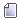###Author Topic: [Source code] D3D Custom Font crash fix.  (Read 257 times)

####VVish##### [Source code] D3D Custom Font crash fix.
« on: March 25, 2019, 12:06:01 am »
Major error caused by unsafe code in the function that was creating texture for black border around symbols in the fonts was finally fixed. BufferOverflow is no more, at least in those particular functions.

This fix is for people who were crashing in D3D clients while using custom fonts (like HP font from PureEvil).

Native:

Code: [Select]
`for (uint y = 0; y < bh; y++)        for (uint x = 0; x < bw; x++)            if (SURF_POINT(lr, x, y))                for (int xx = -1; xx <= 1; xx++)                    for (int yy = -1; yy <= 1; yy++)                        if (!SURF_POINT(lrb, x + xx, y + yy))                            SURF_POINT(lrb, x + xx, y + yy) = 0xFF000000;`
->

Fix:
Code: [Select]
`for (uint y = 0; y < bh; y++)    {        for (uint x = 0; x < bw; x++)        {            if (SURF_POINT(lr, x, y))            {                for (int xx = -1; xx <= 1; xx++)                {                    if (x == 0 && xx == -1) continue;                    if (x + xx >= bw) break;                    for (int yy = -1; yy <= 1; yy++)                    {                        if (y == 0 && yy == -1) continue;                        if (y + yy >= bh) break;                        if (!SURF_POINT(lrb, x + xx, y + yy))                        {                            SURF_POINT(lrb, x + xx, y + yy) = 0xFF000000;                        }                    }                }            }        }    }`
Authors of the solution:
Sacred Cracker,
Vice Dice.